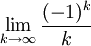# A Motivating Problem for the Alternating Series Test

Previous: The Alternating Series Test

Next: The Alternating Series Test

## Example

Does the following seriesconverge? First let's explore this problem using the tools we already have to see why a new convergence test is needed. This will demonstrate of how, in practice, determining whether an infinite series converges is not a matter of applying a set of algorithms that can be memorized. Rather, we often need to try different approaches and sometimes develop new approaches to solve a given problem.

## Why Do We Need Another Convergence Test?

We've covered the divergence dest and the integral test. Does the divergence test tell us if the series converges? Applying the divergence test, we find that the limitequals zero. The divergence test yields no information, and we must use another test.

Can we apply the integral test? The series have negative and positive values:But the integral test can only applied on a sequence if all of its terms are positive. The integral test yields no information, and so we must use another test.

In this lesson we introduce a convergence test that can be used to establish that this series converges: the alternating series test.

## Investigating the Partial Sums

Remember that for a series to converge, we must have that its partial sums tend to zero. In our example, this means thattends to zero as N tends to infinity. Calculating the first 10 partial sums of our series, for N from 1 to 10, we obtain the values presented in the table below (numbers rounded to the first two decimal places).

k ak=1/k sN
1 -1.00 -1.00
2 0.50 -0.50
3 -0.33 -0.83
4 0.25 -0.58
5 -0.20 -0.78
6 0.17 -0.62
7 -0.14 -0.76
8 0.13 -0.63
9 -0.11 -0.75
10 0.10 -0.65
11 -0.09 -0.74
12 0.08 -0.65
13 -0.08 -0.73
14 0.07 -0.66

It appears that the terms of the sequence are tending towards zero, but does it appear that the partial sums converging? They could be, but our table doesn't prove that the sequence converges to zero. We need a better way to determine whether the series converges. We need a new convergence test.

The alternating series test, applicable to series whose terms alternate between positive and negative, can be applied here. We will next give the alternating series test, and then apply it to show that this series does converge.

Previous: The Alternating Series Test

Next: The Alternating Series Test